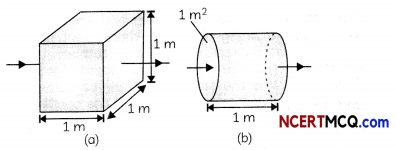## Factors on Which Resistance of a Conductor Depends

Consider a wire of length L, area of cross-section A and having a resistance R. Then the resistance of the wire directly proportional to the length of the wire and inversely proportional to the area of cross-section of the wire, i.e.,
R ∝ L and R ∝ 1/A, or
R = ρL/A

Example 1.
Will current flow more easily through a thick wire or a thin wire of the same material, when connected to the same source? Why?
Current will flow more easily through a thick wire as a thick wire has a greater area of cross section and hence less resistance. We know that resistance is directly proportional to the area of cross section and therefore resistance of a thick wire will be less which means that current will flow more easily asExample 2.
Let the resistance of an electrical component remains constant while the potential difference across the two ends of the component decreases to half of its former value. What change will occur in the current through it?
Let the resistance of the electrical component be equal to R and potential difference across its two ends be V. Therefore, according to Ohm’s law, V = IR or I = $$\frac{V}{R}$$.
R
Now, if V becomes half or $$\frac{V}{2}$$, resistance remaining same,
then I = $$\frac{V / 2}{R}=\frac{V}{2 R}=\frac{1}{2} \times \frac{V}{R}=\frac{1}{2}$$I
Therefore, current also becomes half.
Resistivity
It is an important property of materials and is defined as the resistance offered by a cube of a material of side 1 m when current flows perpendicular to the opposite faces.It is measured in ohm-m. It is a characteristic property of the material and varies with temperature. Metals and alloys have very low resistivity but insulators have very high resistivity.## Applications of Alloys

Alloys are used in electric heating devices such as electric irons, geysers & toasters for the following reasons:

1. The resistivity of alloys is generally higher than that of pure metals which form the alloy.
2. They do not oxidize readily at high temperatures since resistivity changes less rapidly with changes in temperature.